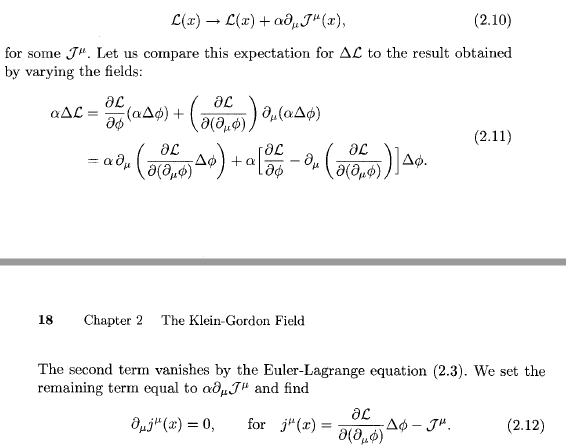# Noether current

LCSphysicist
Homework Statement:
I can't see why the expression gives by the author is right.
Relevant Equations:
.I just don't understand what happened after (2.11). That' is, the second term is zero, so we have
$$\alpha \Delta L = \alpha \partial_{\mu} ( \frac{\partial L}{\partial (\partial_{\mu}\phi)} \Delta \phi )$$
So, second (2.10), isn't ##\Delta L = \alpha \partial_{\mu} J^{\mu}(x)##? So shouldn't the final equation reduce to this?:

##\alpha \alpha \partial_{\mu} J^{\mu}(x) = \alpha \partial_{\mu} ( \frac{\partial L}{\partial (\partial_{\mu}\phi)} \Delta \phi )##
##\partial_{\mu} (\frac{\partial L}{\partial (\partial_{\mu}\phi)} \Delta \phi - \alpha J^{\mu}(x) )##